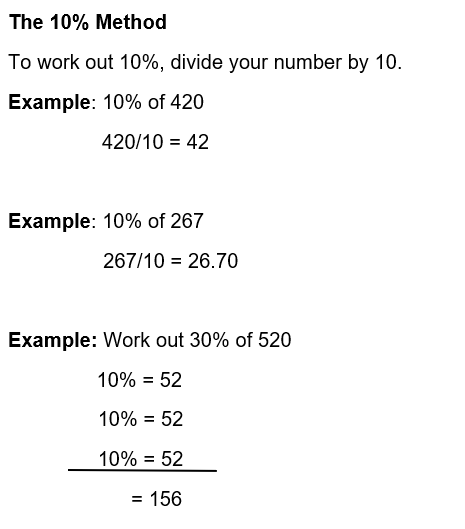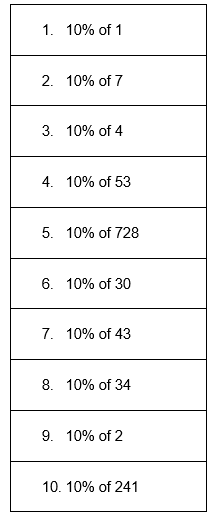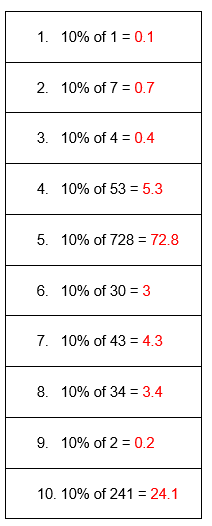# Finding 10 Percent of a Number Worksheet (with answer key + PDF)

The Latin word “per centum,” which means “by the hundred,” is where the word “percentage” originally came from. With 100 as the denominator, percentages are fractions. To put it another way, it’s the relationship between a part and a whole in which the value of the whole is always assumed to be 100.

## What is the “Finding 10 Percent of a Number Worksheet (with answer key + PDF)”?

This worksheet will explore finding 10 percent of a number.

How to find 10 percent of a number?

One-tenth is equal to 10%. Simply divide a number by 10 or move the decimal point one place to the left to calculate 10 percent of a number.

How will the “Finding 10 Percent of a Number Worksheet (with answer key + PDF)” help you?

This worksheet will help you to calculate and find the percentage of the number.

Instructions on how to use the “Finding 10 Percent of a Number Worksheet (with answer key + PDF).”

Study the concept and examples given and try to solve the given exercises below.

## Conclusion

The use of percentages is widespread and diverse. For instance, many statistics in the media, bank interest rates, retail discounts, and inflation rates are all expressed as percentages. For understanding the financial aspects of daily life, percentages are crucial.

If you have any inquiries or feedback, please let us know.

## Finding 10 Percent of a Number Worksheet (with answer key + PDF)

What is the percentage?

The whole value of a percentage is always 100, making it a ratio or fraction. Sam, for instance, would have received 30 out of a possible 100 points if he had received 30% on his math test. In ratio form, it is written as 30:100, and in fraction form as 30/100.

Percentage Definition:

A percentage is defined as a given part or amount in every hundred. It is a fraction with 100 as the denominator and is represented by the symbol “%”.

How to get a Percentage?

Percent is another name for indicating hundredths. Thus, 1% is one-hundredth, which means 1%=1/100=0.01.## Worksheet

Find 10% in each number.